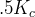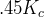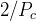# 1 PID Tuning

## 1.1 PID Controller

A proportional-integral-derivative controller (PID controller) is a common feedback loop component in industrial control systems.1

The Controller compares a measured value from a process (typically an industrial process) with a reference set point value. The difference (or "error" signal) is then used to calculate a new value for a manipulable input to the process that brings the process measured value back to its desired set point.

Unlike simpler control algorithms, the PID controller can adjust process outputs based on the history and rate of change of the error signal, which gives more accurate and stable control. (It can be shown mathematically that a PID loop will produce accurate, stable control in cases where a simple proportional control would either have a steady-state error or would cause the process to oscillate).

### 1.1.1 Control loop basics

Intuitively, the PID loop tries to automate what an intelligent operator with a gauge and a control knob would do. The operator would read a gauge showing the output measurement of a process, and use the knob to adjust the input of the process (the "action") until the process's output measurement stabilizes at the desired value on the gauge.

In older control literature this adjustment process is called a "reset" action. The position of the needle on the gauge is a "measurement", "process value" or "process variable". The desired value on the gauge is called a "set point" (also called "set value"). The difference between the gauge's needle and the set point is the "error".

A control loop consists of three parts:

1. Measurement by a sensor connected to the process (e.g. encoder),

2. Decision in a controller element,

3. Action through an output device such as an motor.

As the controller reads a sensor, it subtracts this measurement from the "set point" to determine the "error". It then uses the error to calculate a correction to the process's input variable (the "action") so that this correction will remove the error from the process's output measurement.

In a PID loop, correction is calculated from the error in three ways: cancel out the current error directly (Proportional), the amount of time the error has continued uncorrected (Integral), and anticipate the future error from the rate of change of the error over time (Derivative).

A PID controller can be used to control any measurable variable which can be affected by manipulating some other process variable. For example, it can be used to control temperature, pressure, flow rate, chemical composition, speed, or other variables. Automobile cruise control is an example of a process outside of industry which utilizes crude PID control.

Some control systems arrange PID controllers in cascades or networks. That is, a "master" control produces signals used by "slave" controllers. One common situation is motor controls: one often wants the motor to have a controlled speed, with the "slave" controller (often built into a variable frequency drive) directly managing the speed based on a proportional input. This "slave" input is fed by the "master" controller's output, which is controlling based upon a related variable.

### 1.1.2 Theory

"PID" is named after its three correcting calculations, which all add to and adjust the controlled quantity. These additions are actually "subtractions" of error, because the proportions are usually negative:

##### Proportional

To handle the present, the error is multiplied by a (negative) constant P (for "proportional"), and added to (subtracting error from) the controlled quantity. P is only valid in the band over which a controller's output is proportional to the error of the system. Note that when the error is zero, a proportional controller's output is zero.

##### Integral

To learn from the past, the error is integrated (added up) over a period of time, and then multiplied by a (negative) constant I (making an average), and added to (subtracting error from) the controlled quantity. I averages the measured error to find the process output's average error from the set point. A simple proportional system either oscillates, moving back and forth around the set point because there's nothing to remove the error when it overshoots, or oscillates and/or stabilizes at a too low or too high value. By adding a negative proportion of (i.e. subtracting part of) the average error from the process input, the average difference between the process output and the set point is always being reduced. Therefore, eventually, a well-tuned PID loop's process output will settle down at the set point.

##### Derivative

To handle the future, the first derivative (the slope of the error) over time is calculated, and multiplied by another (negative) constant D, and also added to (subtracting error from) the controlled quantity. The derivative term controls the response to a change in the system. The larger the derivative term, the more rapidly the controller responds to changes in the process's output.

More technically, a PID loop can be characterized as a filter applied to a complex frequency-domain system. This is useful in order to calculate whether it will actually reach a stable value. If the values are chosen incorrectly, the controlled process input can oscillate, and the process output may never stay at the set point.

### 1.1.3 Loop Tuning

"Tuning" a control loop is the adjustment of its control parameters (gain/proportional band, integral gain/reset, derivative gain/rate) to the optimum values for the desired control response. The optimum behavior on a process change or set point change varies depending on the application. Some processes must not allow an overshoot of the process variable from the set point. Other processes must minimize the energy expended in reaching a new set point. Generally stability of response is required and the process must not oscillate for any combination of process conditions and set points.

Tuning of loops is made more complicated by the response time of the process; it may take minutes or hours for a set point change to produce a stable effect. Some processes have a degree of non-linearity and so parameters that work well at full-load conditions don't work when the process is starting up from no-load. This section describes some traditional manual methods for loop tuning.

There are several methods for tuning a PID loop. The choice of method will depend largely on whether or not the loop can be taken "offline" for tuning, and the response speed of the system. If the system can be taken offline, the best tuning method often involves subjecting the system to a step change in input, measuring the output as a function of time, and using this response to determine the control parameters.

##### Simple method

If the system must remain on line, one tuning method is to first set the I and D values to zero. Increase the P until the output of the loop oscillates. Then increase I until oscillation stops. Finally, increase D until the loop is acceptably quick to reach its reference. A fast PID loop tuning usually overshoots slightly to reach the set point more quickly; however, some systems cannot accept overshoot.

 Parameter Rise Time Overshoot Settling Time S.S. Error P Decrease Increase Small Change Decrease I Decrease Increase Increase Eliminate D Small Change Decrease Decrease Small Change

Effects of increasing parameters

##### Ziegler-Nichols method

Another tuning method is formally known as the "Ziegler-Nichols method", introduced by John G. Ziegler and Nathaniel B. Nichols. It starts in the same way as the method described before: first set the I and D gains to zero and then increase the P gain and expose the loop to external interference for example knocking the motor axis to cause it to move out of equilibrium in order to determine critical gain and period of oscillation until the output of the loop starts to oscillate. Write down the critical gain () and the oscillation period of the output (). Then adjust the P, I and D controls as the table shows:

 Control type P I D PPIPID##### Final Steps

After tuning the axis check the following error with Hal Scope to make sure it is within your machine requirements. More information on Hal Scope is in the HAL User manual.

## Footnotes

1   This Subsection is taken from an much more extensive article found at http://en.wikipedia.org/wiki/PID_controller back